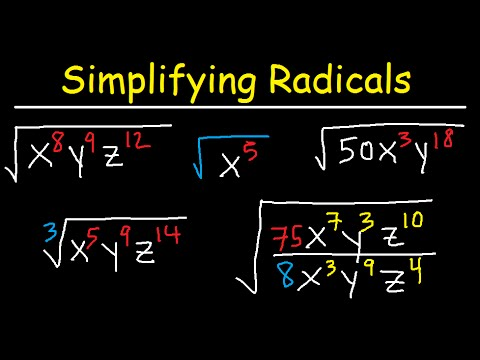# Simplifying Radicals With Variables, Exponents, Fractions, Cube Roots - Algebra

##By The Organic Chemistry Tutor

This algebra 1 & 2 video tutorial shows you how to simplify radicals with variables, fractions, and exponents that contains both square roots, cube roots, and variables such as x, y, and z. This video contains plenty of examples and practice problems.Here is a list of topics: 1. Simplifying Radical Expressions With Variables & Exponents 2. Simplifying Radicals With Fractions & Variables 3. Radicals With Square Roots & Cube Roots 4. Radical Expressions with X, Y, and Z 5. Absolute Value - Simplifying radical expressions# Math Antics - The Pythagorean Theorem

##By mathantics